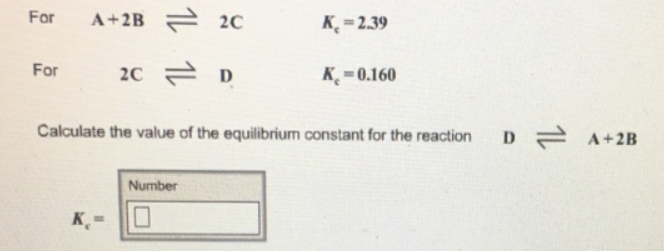# Problem: For A + 2B ⇌ 2C           Kc = 2.39For 2C ⇌ D            Kc = 0.160Calculate the value of the equilibrium constant for the reactionD ⇌ A + 2B

###### FREE Expert Solution
91% (243 ratings)###### Problem Details

For A + 2B ⇌ 2C           Kc = 2.39

For 2C ⇌ D            Kc = 0.160

Calculate the value of the equilibrium constant for the reaction

D ⇌ A + 2B